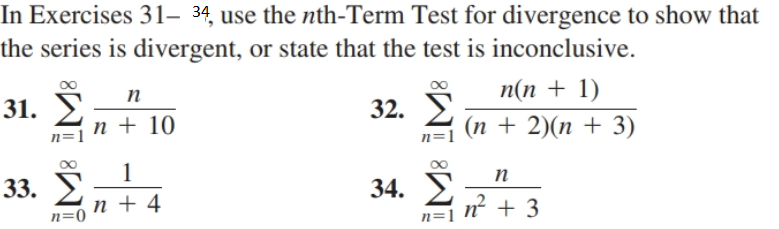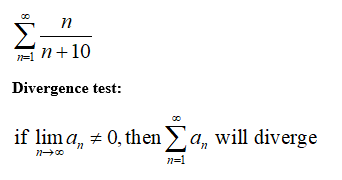# In Exercises 31– 34, use the nth-Term Test for divergence to show thatthe series is divergent, or state that the test is inconclusive.п(n + 1)(п + 2)(п + 3)п31. En + 10n=132. En=133. Eп34. Eп+ 4n=0n + 3n=1

Question
1 viewshelp_outlineImage TranscriptioncloseIn Exercises 31– 34, use the nth-Term Test for divergence to show that the series is divergent, or state that the test is inconclusive. п(n + 1) (п + 2)(п + 3) п 31. E n + 10 n=1 32. E n=1 33. E п 34. E п+ 4 n=0 n + 3 n=1 fullscreen
check_circle

Step 1

Hi there, the exact one was not specified. so, I have solved first question.

Step 2

To show that the given series is diverge.

Step 3

Given information:...

### Want to see the full answer?

See Solution

#### Want to see this answer and more?

Solutions are written by subject experts who are available 24/7. Questions are typically answered within 1 hour.*

See Solution
*Response times may vary by subject and question.
Tagged in